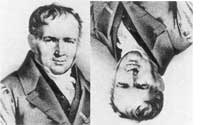This morning,
Michel Van den Bergh
posted an interesting paper on the arXiv
entitled Double
Poisson Algebras
. His main motivation was the construction of a
natural Poisson structure on quotient varieties of representations of
deformed multiplicative preprojective algebras (introduced by
Crawley-Boevey and Shaw in Multiplicative
preprojective algebras, middle convolution and the Deligne-Simpson
problem
) which he achieves by extending his double Poisson structure
on the path algebra of the quiver to the 'obvious' universal
localization, that is the one by inverting all $1+aa^{\star}$ for $a$ an
arrow and $a^{\star}$ its double (the one in the other direction).
For me the more interesting fact of this paper is that his double
bracket on the path algebra of a double quiver gives finer information
than the _necklace Lie algebra_ as defined in my (old) paper with Raf
Bocklandt Necklace
Lie algebras and noncommutative symplectic geometry
. I will
certainly come back to this later when I have more energy but just to
wet your appetite let me point out that Michel calls a _double bracket_
on an algebra $A$ a bilinear map
$\{ \{ -,- \} \}~:~A \times A \rightarrow A \otimes A$
which is a derivation in the _second_
argument (for the outer bimodulke structure on $A$) and satisfies
$\{ \{ a,b \} \} = – \{ \{ b,a \} \}^o$ with $~(u \otimes v)^0 = v \otimes u$
Given such a double bracket one can define an ordinary
bracket (using standard Hopf-algebra notation)
$\{ a,b \} = \sum \{ \{ a,b \} \}_{(1)} \{ \{ a,b \} \}_{(2)}$
which makes $A$ into
a Loday
algebra
and induces a Lie algebra structure on $A/[A,A]$. He then
goes on to define such a double bracket on the path algebra of a double
quiver in such a way that the associated Lie structure above is the
necklace Lie algebra.

### Similar Posts:

Published in featured